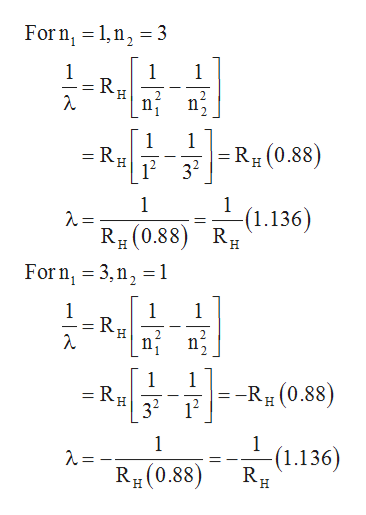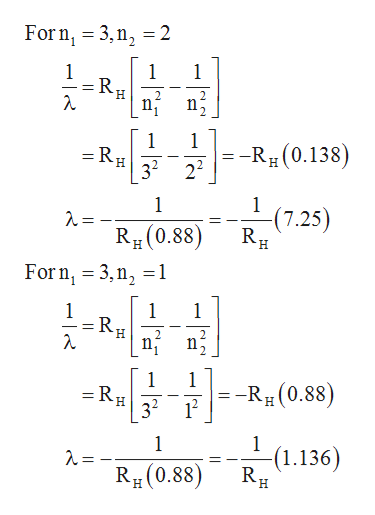# The electron in an (unbound) hydrogen atom (H(g)) is excited from the ground state to the n=3 state.Which of the following statements are true and which are false.The wavelength of the light emitted when the electron returns to the ground state from n=3 is the same as the wavelength of light absorbed to go from n=1 to n=3.The wavelength of light emitted when the electron drops from n=3 to n=2 is shorter than the wavelength of light emitted if the electron falls from n=3 to n=1.The first excited state corresponds to n=2. The electron is farther from the nucleus on average in the n=3 state than in the ground state. It takes more energy to ionize the electron from n=3 than it does from the ground state.

Question
3 views

The electron in an (unbound) hydrogen atom (H(g)) is excited from the ground state to the n=3 state.
Which of the following statements are true and which are false.

The wavelength of the light emitted when the electron returns to the ground state from n=3 is the same as the wavelength of light absorbed to go from n=1 to n=3.

The wavelength of light emitted when the electron drops from n=3 to n=2 is shorter than the wavelength of light emitted if the electron falls from n=3 to n=1.

The first excited state corresponds to n=2.

The electron is farther from the nucleus on average in the n=3 state than in the ground state.

It takes more energy to ionize the electron from n=3 than it does from the ground state.

check_circle

Step 1

The Rydberg equation is given below.

Step 2

The wavelength for both the cases can be calculated as follows,help_outlineImage TranscriptioncloseForn 1n2 3 1 1 R H n2 1 1 R(0.88) H 12 32 1 1 (1.136 R (0.88 R Forn 3,n2 1 1 1 1 2 1 1 --R (0.88) 32 12 1 -(1.136) RB(0.88) H fullscreen
Step 3

The magnitude of wavelength in both the cases is same. Thus the given statement is true.

...help_outlineImage TranscriptioncloseForn 3,n 2 1 1 1 H 1 =Rg 32 1 -Rg(0.138) 22 1 1 (7.25) RB(0.88) H H Forn, 3,n2 1 1 1 1 2 1 =RH 1 --Rg(0.88) 32 1 1 (1.136) RB(0.88) H H fullscreen

### Want to see the full answer?

See Solution

#### Want to see this answer and more?

Solutions are written by subject experts who are available 24/7. Questions are typically answered within 1 hour.*

See Solution
*Response times may vary by subject and question.
Tagged in

### Chemistry